# Circuits Circuits We will build circuits for addition

• Slides: 65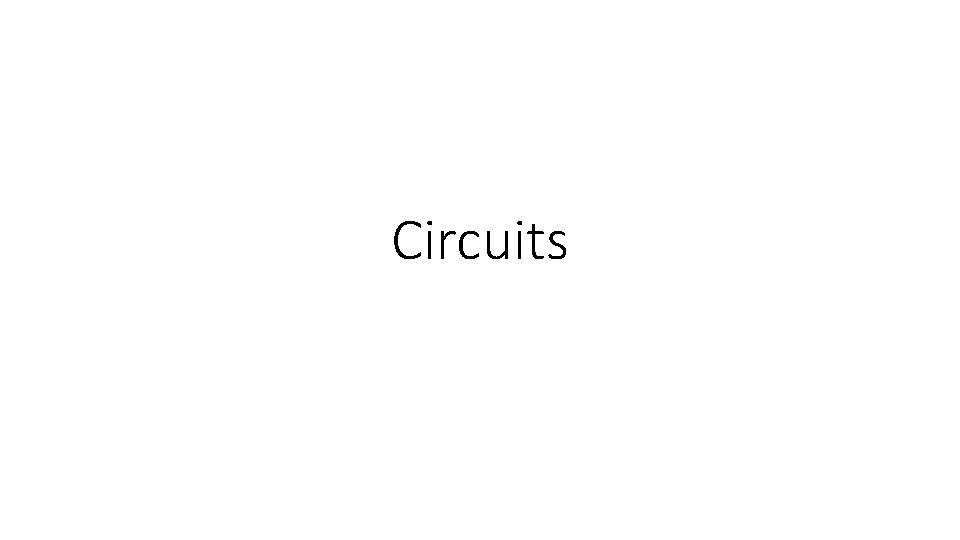CircuitsCircuits • We will build circuits for addition, multiplication and other arithmetic operations. • This is actually used! Everywhere!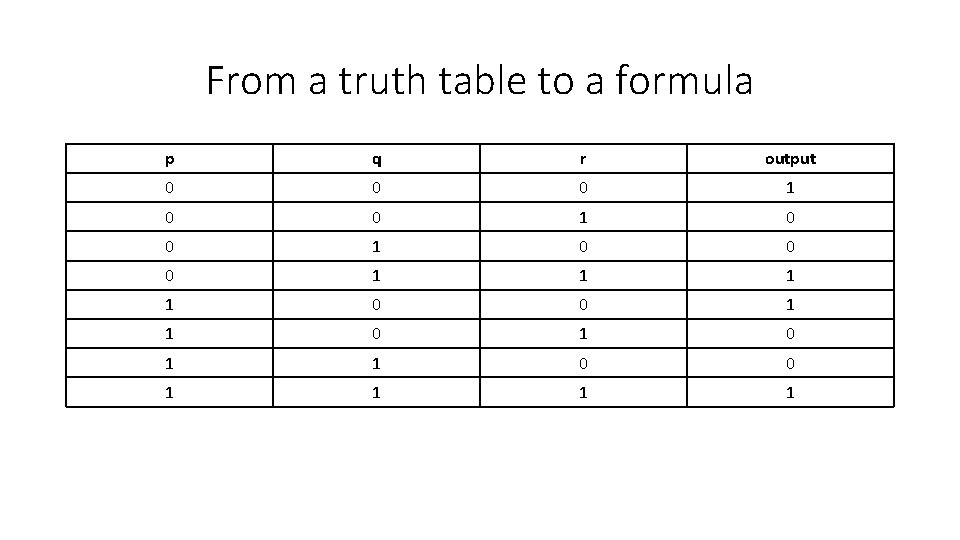From a truth table to a formula p q r output 0 0 0 1 0 0 0 1 1 0 1 1 0 0 1 1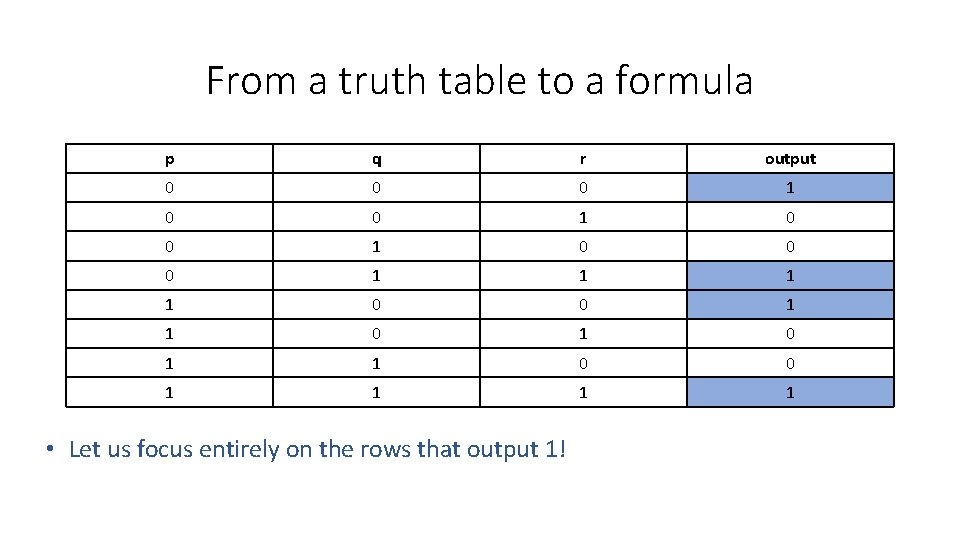From a truth table to a formula p q r output 0 0 0 1 0 0 0 1 1 0 1 1 0 0 1 1 • Let us focus entirely on the rows that output 1!p q r output 0 0 0 1 0 0 0 1 1 0 1 1 0 0 1 1 Focusing on the st 1 row… p q r output 0 0 0 1 • Write a formula that is ’ 1’ only on inputs p =0, q = 0, r = 0.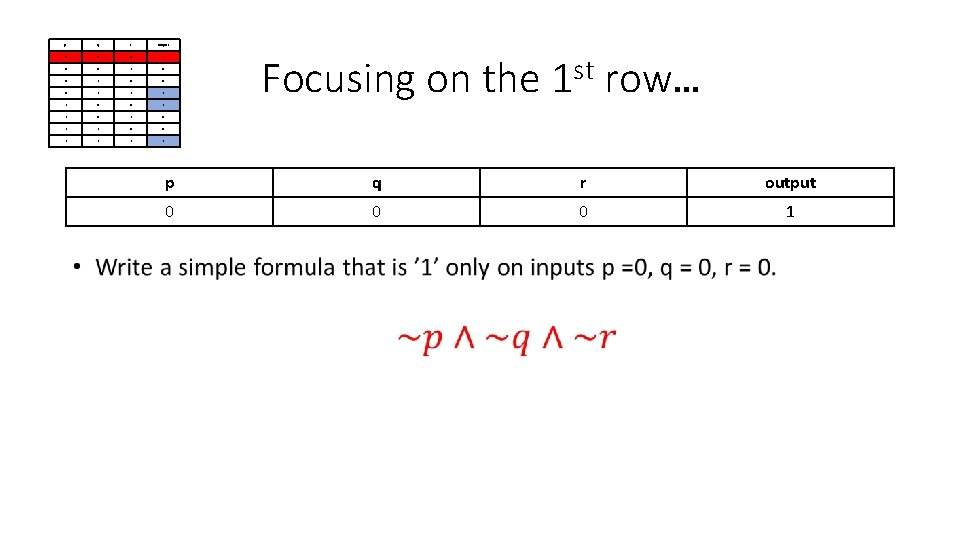p q r output 0 0 0 1 0 0 0 1 1 0 1 1 0 0 1 1 Focusing on the st 1 row… p q r output 0 0 0 1p q r output 0 0 0 1 0 0 0 1 1 0 1 1 0 0 1 1 Focusing on the th 4 row… p q r output 0 1 1 1 • Same dealp q r output 0 0 0 1 0 0 0 1 1 0 1 1 0 0 1 1 Focusing on the th 4 row… p q r output 0 1 1 1 • Same deal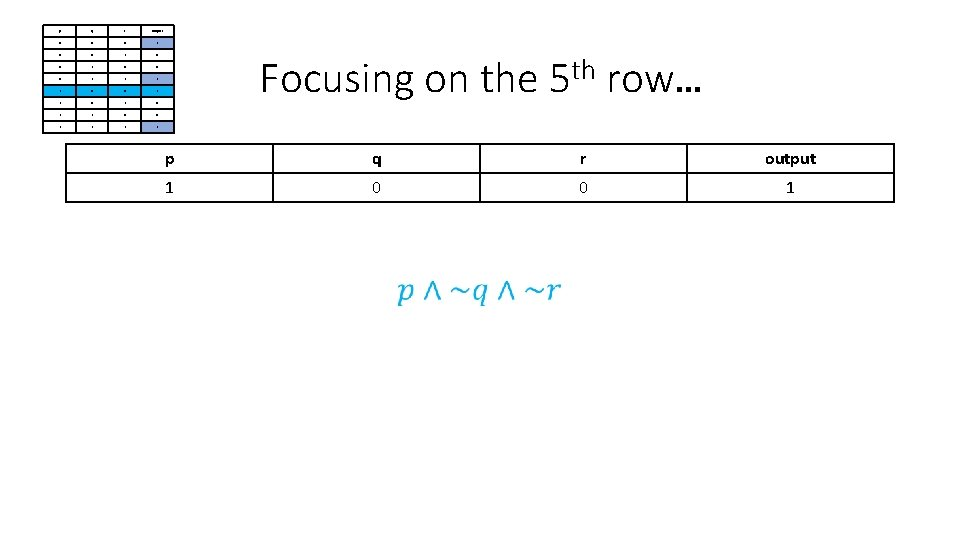p q r output 0 0 0 1 0 0 0 1 1 0 1 1 0 0 1 1 Focusing on the th 5 row… p q r output 1 0 0 1p q r output 0 0 0 1 0 0 0 1 1 0 1 1 0 0 1 1 Focusing on the th 8 row… p q r output 1 1How do we combine those simple formulae?How do we combine those simple formulae? p q r output 0 0 0 1 0 0 0 1 1 0 1 1 0 0 1 1 • Outputs 1 if and only if the truth table outputs 1!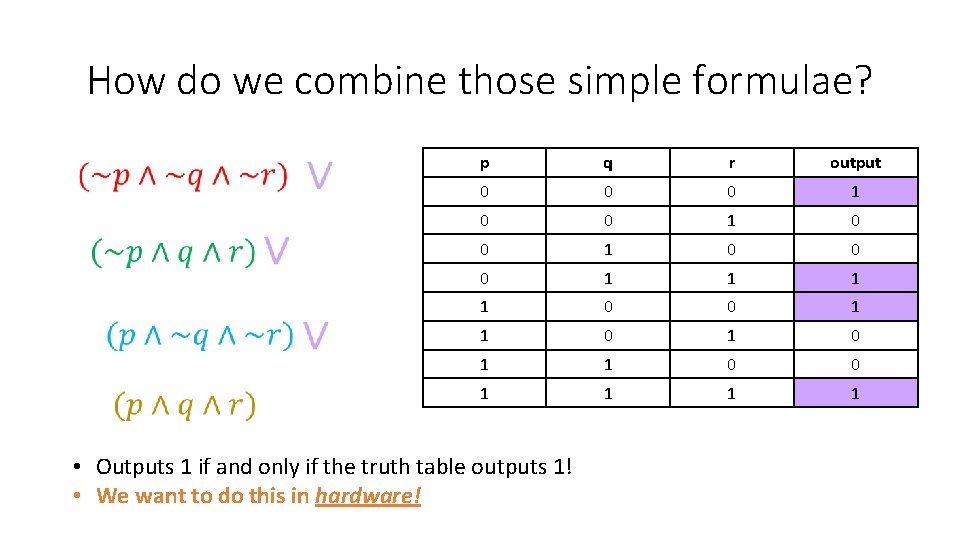How do we combine those simple formulae? p q r output 0 0 0 1 0 0 0 1 1 0 1 1 0 0 1 1 • Outputs 1 if and only if the truth table outputs 1! • We want to do this in hardware!Logical gates • The smallest pieces of hardware that we will examine are called logical gates. • Every gate that we encounter will take bits as inputs and will emit one bit as output. Gate • Those gates can connect to each other in various different ways in order to create more complex circuits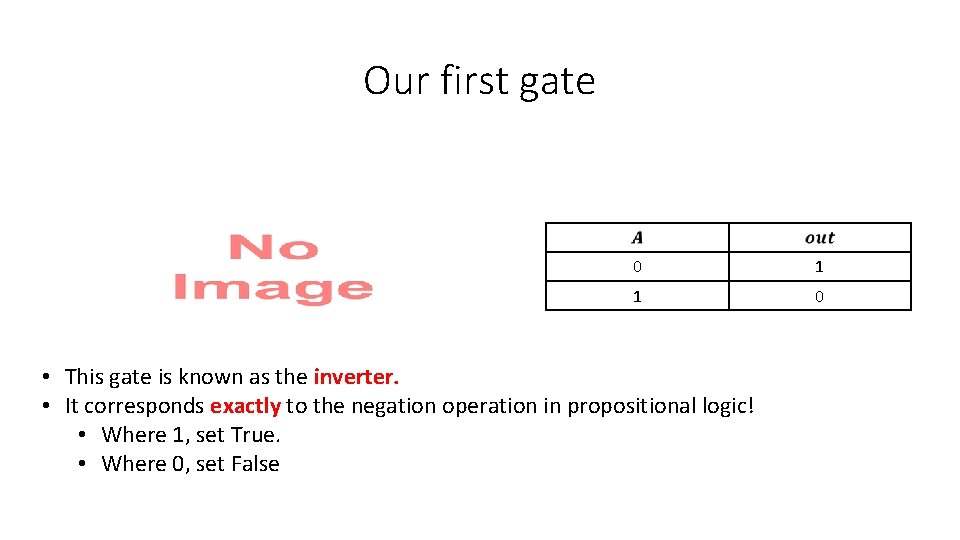Our first gate 0 1 1 0 • This gate is known as the inverter. • It corresponds exactly to the negation operation in propositional logic! • Where 1, set True. • Where 0, set False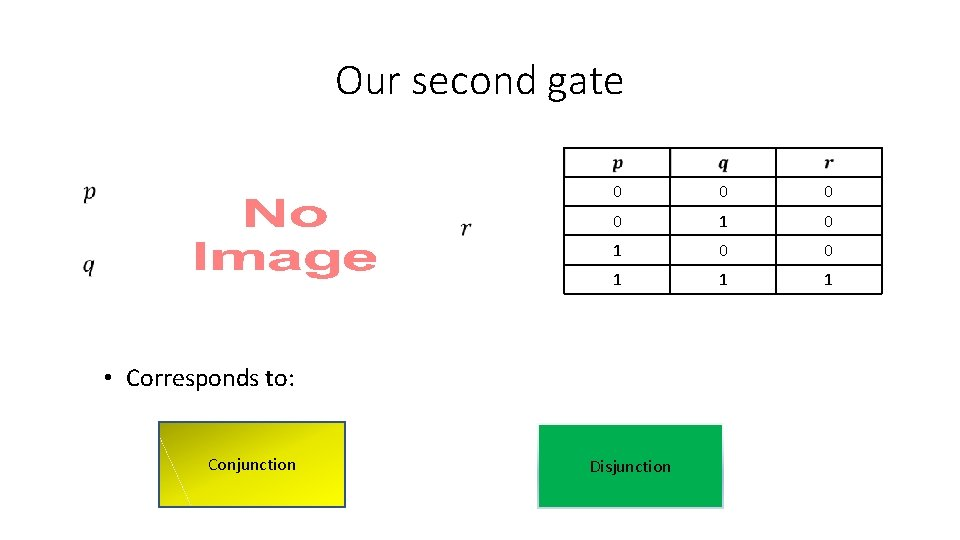Our second gate 0 0 1 1 1 • Corresponds to: Conjunction Disjunction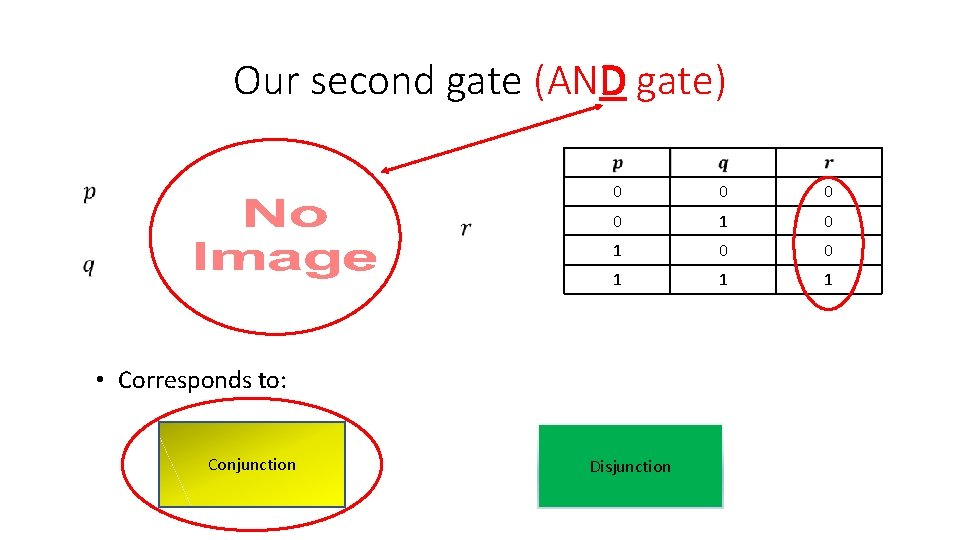Our second gate (AND gate) 0 0 1 1 1 • Corresponds to: Conjunction DisjunctionOur third gate (OR gate) • Corresponds to logical disjunction (OR) 0 0 1 1 1 0 1 1Exercises • For the following logical circuits, vote on the Boolean functions of their inputs to which they correspond.Exercises • For the following logical circuits, vote on the Boolean functions of their inputs to which they correspond.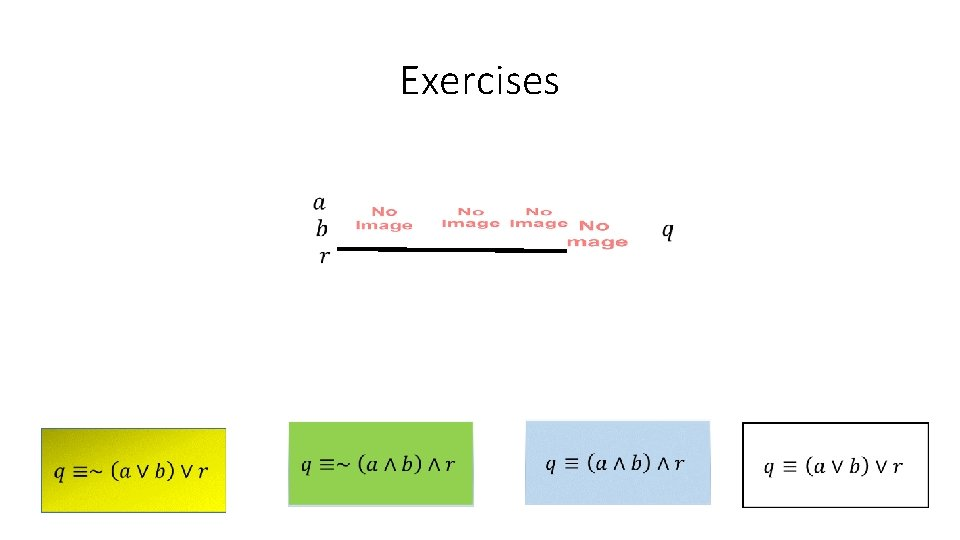Exercises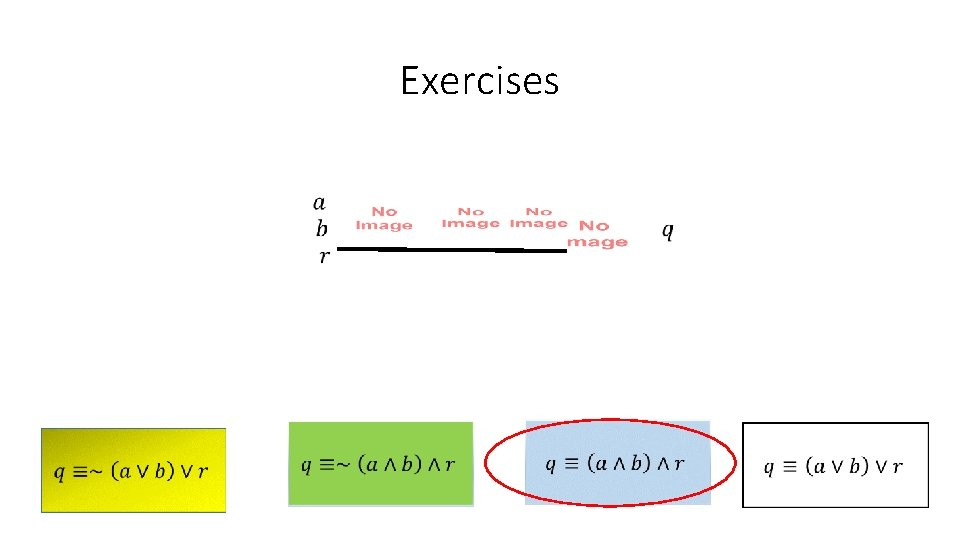Exercises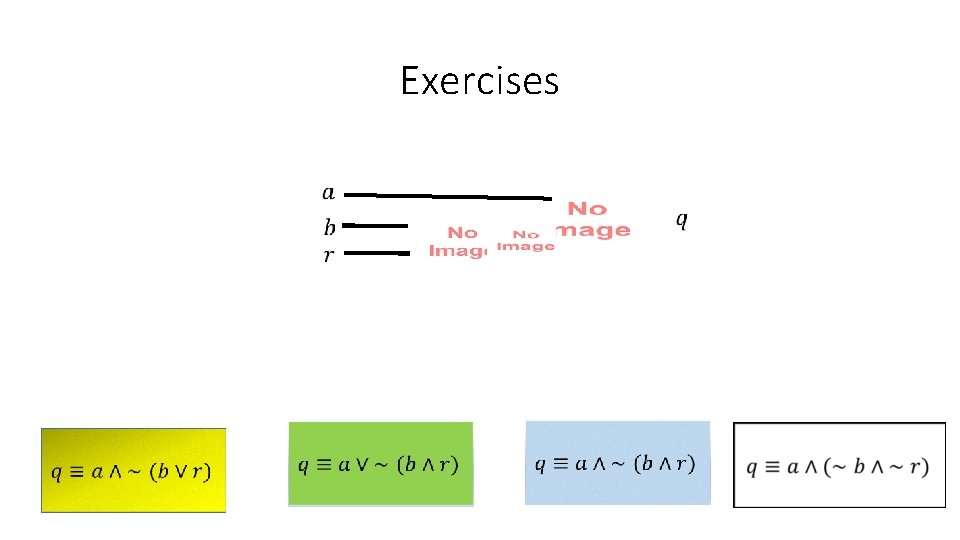Exercises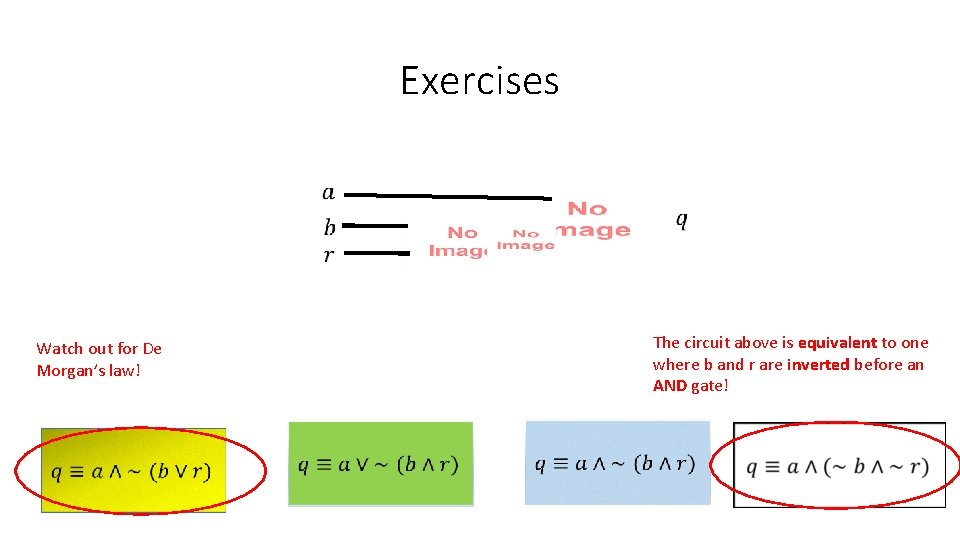Exercises The circuit above is equivalent to one where b and r are inverted before an AND gate! Watch out for De Morgan’s law!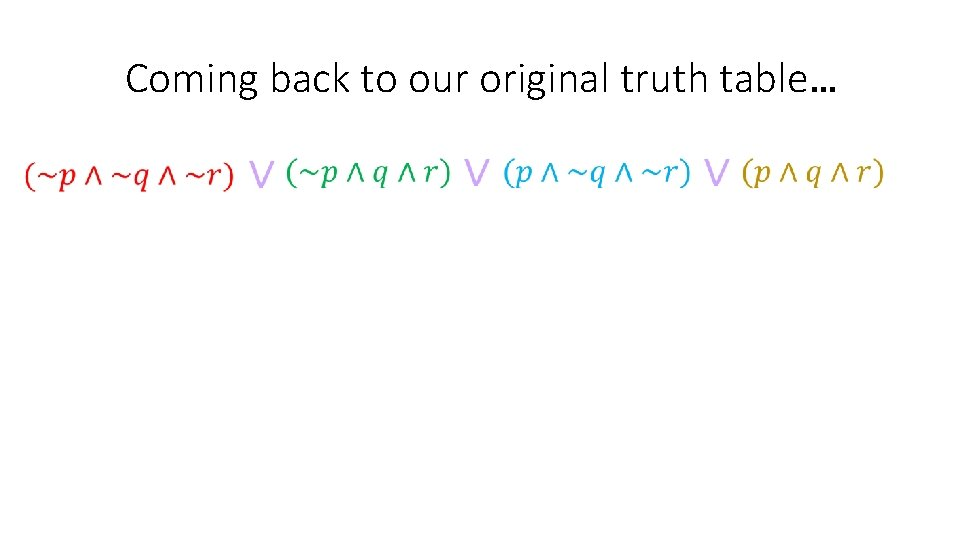Coming back to our original truth table…Coming back to our original truth table… • For each small formula we have a circuit, and we will combine with a 4 -input OR gate!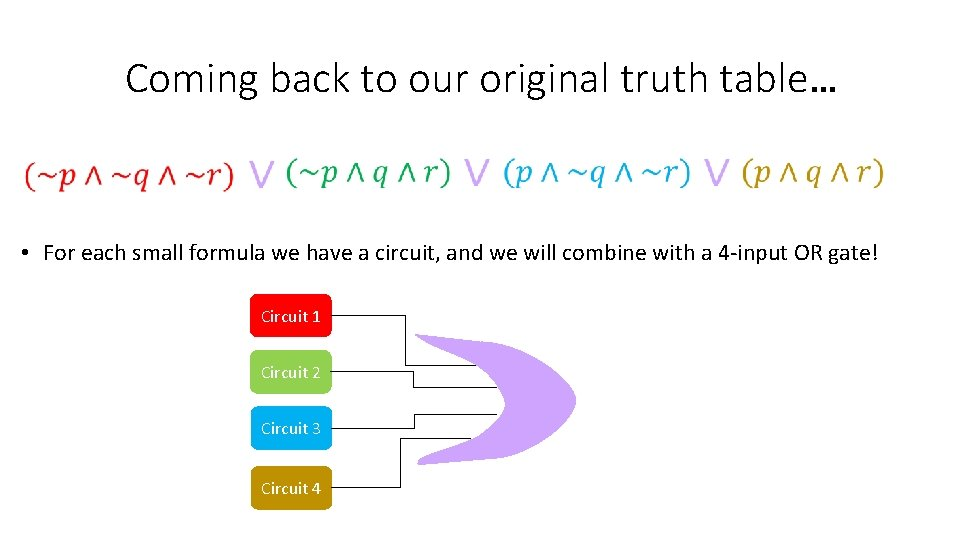Coming back to our original truth table… • For each small formula we have a circuit, and we will combine with a 4 -input OR gate! Circuit 1 Circuit 2 Circuit 3 Circuit 4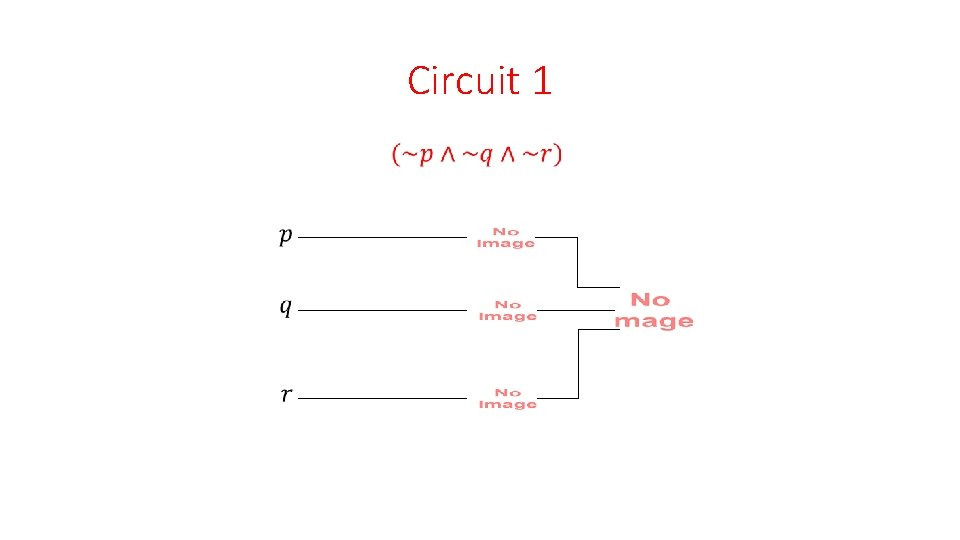Circuit 1Circuit 2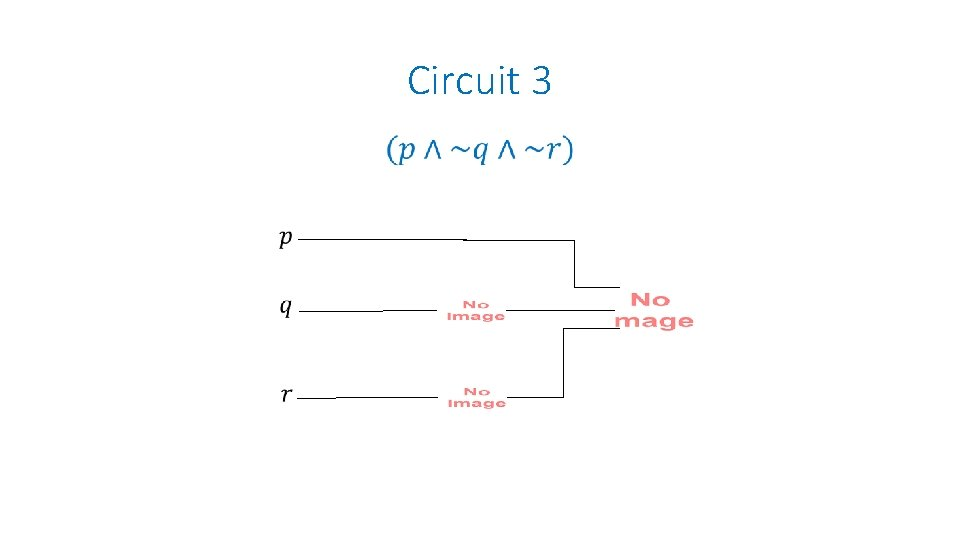Circuit 3Circuit 4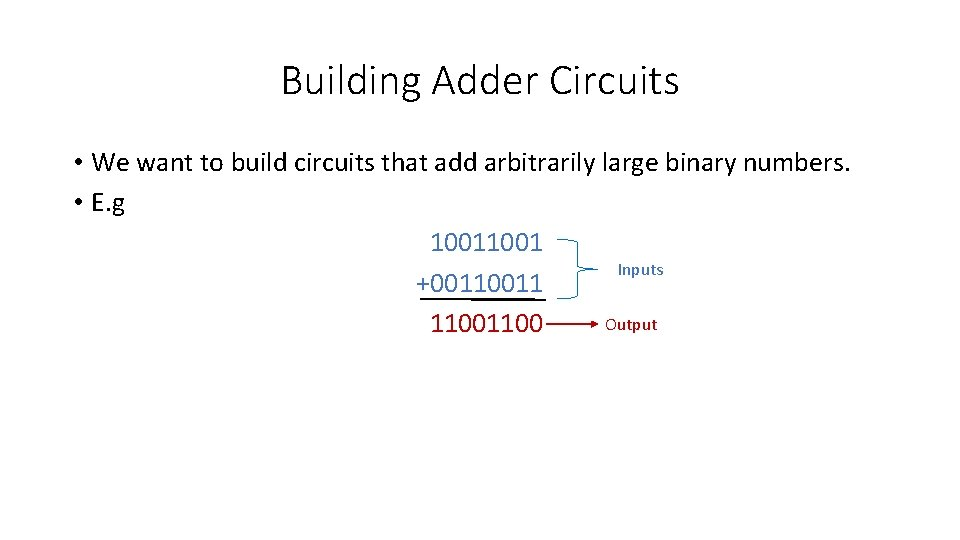Building Adder Circuits • We want to build circuits that add arbitrarily large binary numbers. • E. g 1001 Inputs +0011 Output 1100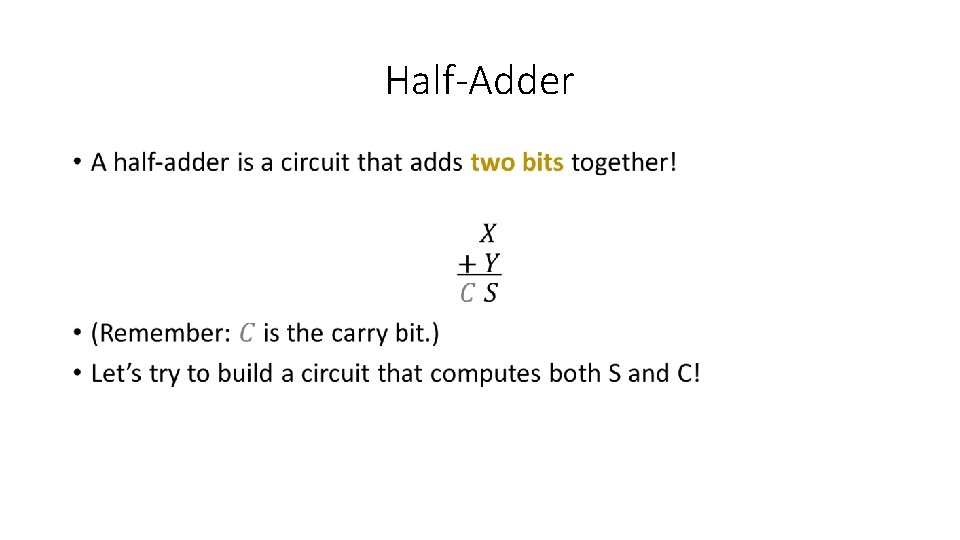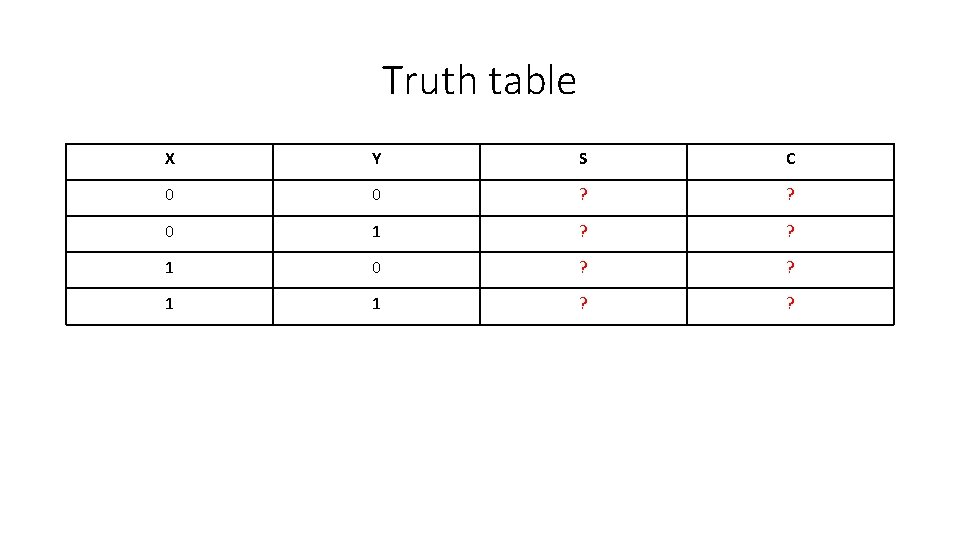Truth table X Y S C 0 0 ? ? 0 1 ? ? 1 0 ? ? 1 1 ? ?Truth table X Y S C 0 0 0 1 1 0 1 0 1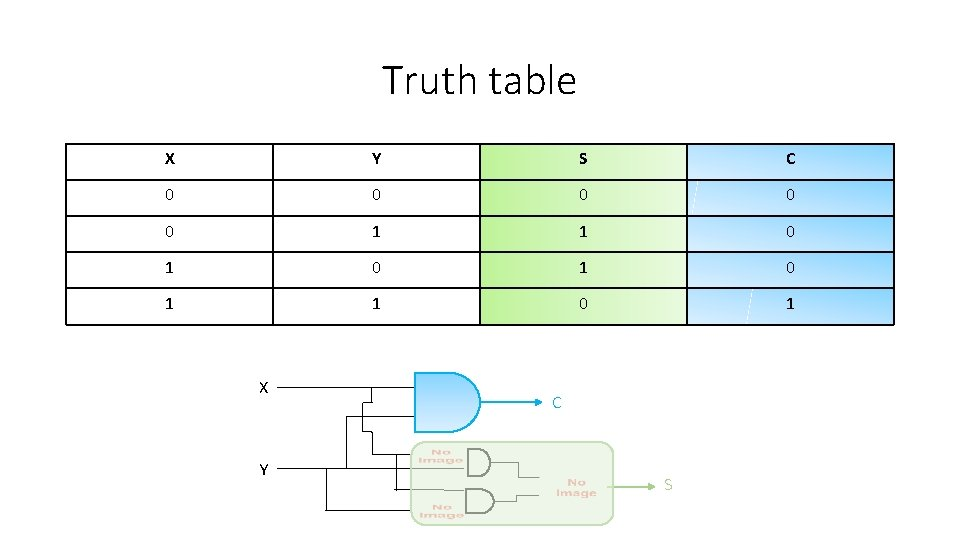Truth table X Y S C 0 0 0 1 1 0 1 0 1 X Y C S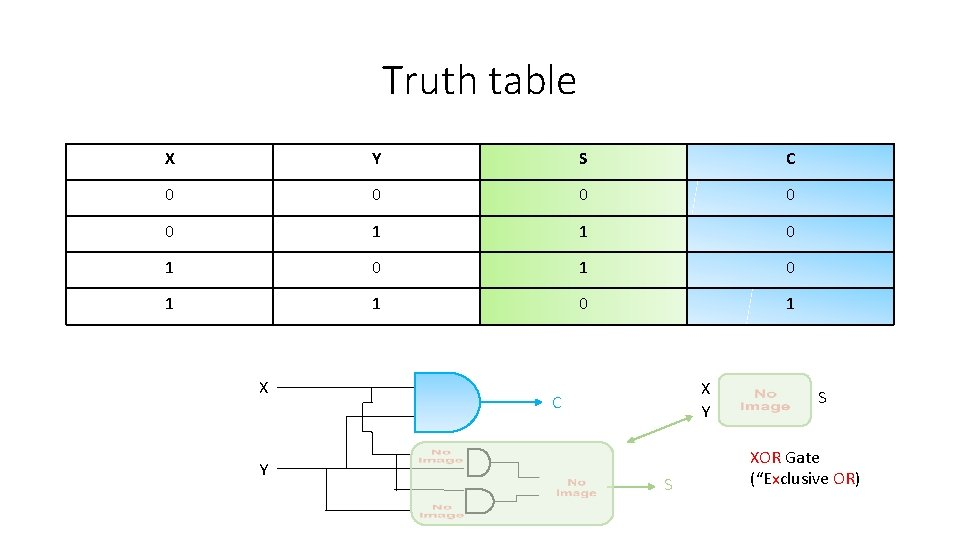Truth table X Y S C 0 0 0 1 1 0 1 0 1 X Y C S S XOR Gate (“Exclusive OR)Half-Adder Abstraction: We can now consider the half-adder as a black box that receives two inputs and emits two outputs.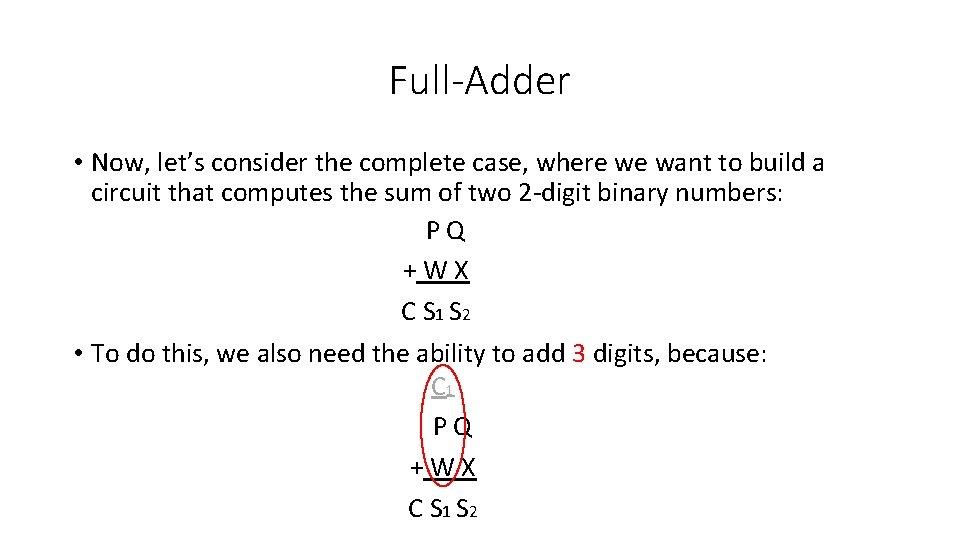Full-Adder • Now, let’s consider the complete case, where we want to build a circuit that computes the sum of two 2 -digit binary numbers: P Q + W X C S 1 S 2 • To do this, we also need the ability to add 3 digits, because: C 1 P Q + W X C S 1 S 2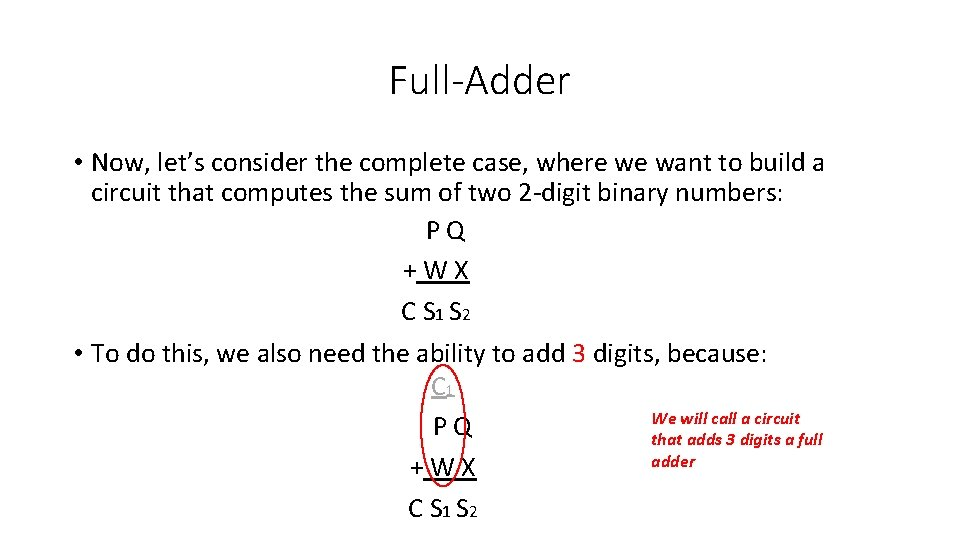Full-Adder • Now, let’s consider the complete case, where we want to build a circuit that computes the sum of two 2 -digit binary numbers: P Q + W X C S 1 S 2 • To do this, we also need the ability to add 3 digits, because: C 1 We will call a circuit P Q that adds 3 digits a full adder + W X C S 1 S 2We could do the truth table…. P Q + W X C S 1 S 2 P Q W X 0 0 0 0 1 1 0 0 0 1 0 1 1 0 0 0 1 1 0 1 0 1 1 0 0 1 1 1 1 0 1 1 C S 1 S 2We could do the truth table…. P Q + W X C S 1 S 2 P Q W X 0 0 0 0 1 1 0 0 0 1 0 1 1 0 0 0 1 1 0 1 0 1 1 0 0 1 1 1 1 0 1 1 C S 1 S 2 But it’s time consuming and we are all busy people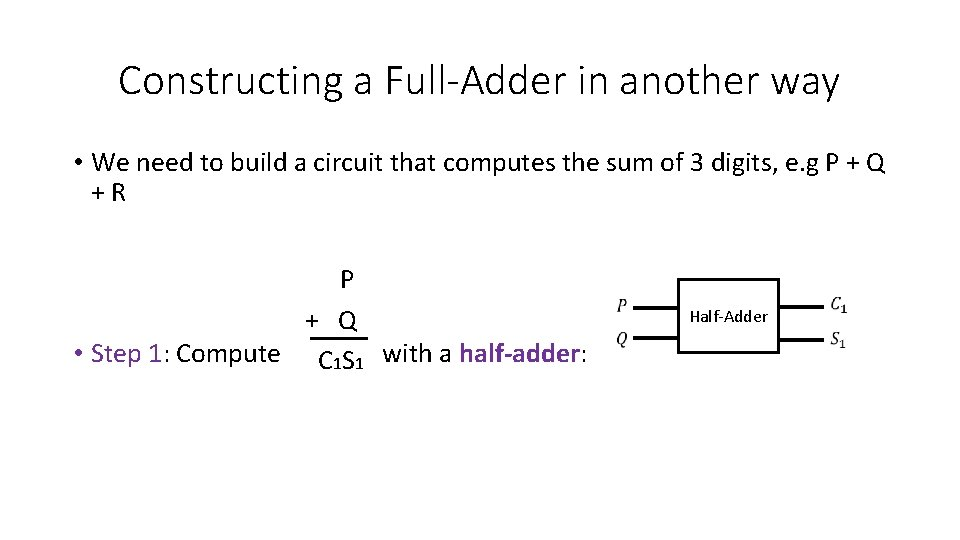Constructing a Full-Adder in another way • We need to build a circuit that computes the sum of 3 digits, e. g P + Q + R P + Q • Step 1: Compute with a half-adder: C 1 S 1 Half-Adder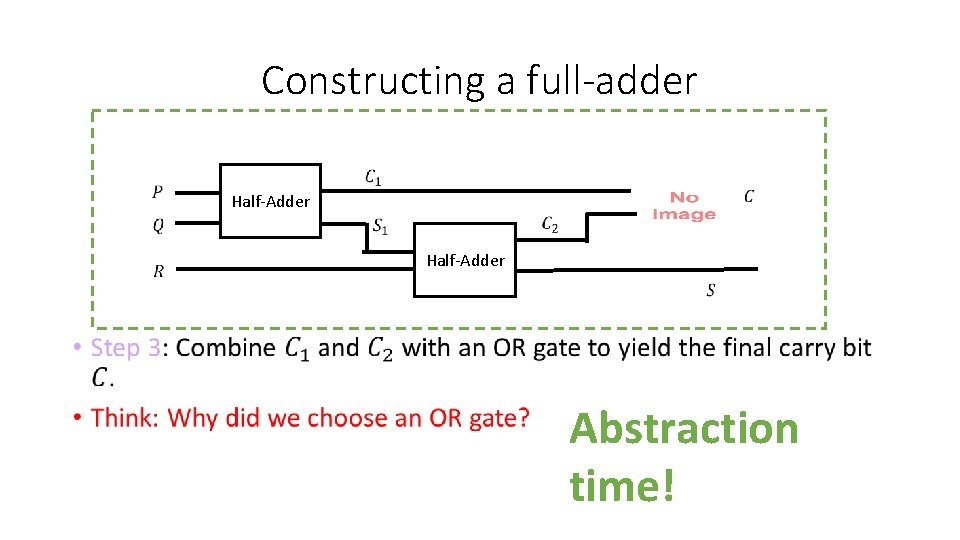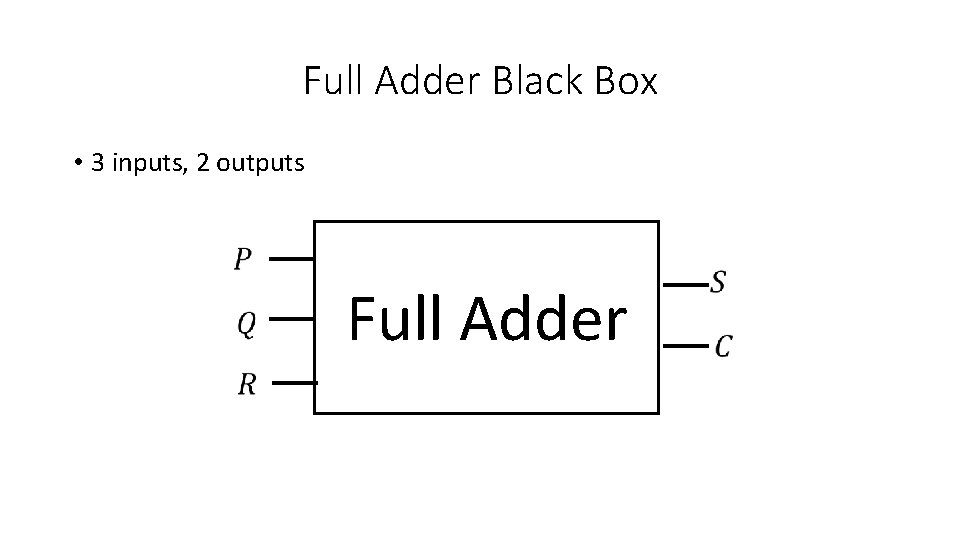2 -bit adder • However, we still have not solved our original problem, which is to construct a circuit that adds 2 -bit numbers! P Q + W X C S 1 S 2 • So, we need a circuit that takes 4 inputs and emits 3 outputs: 2 -bit adder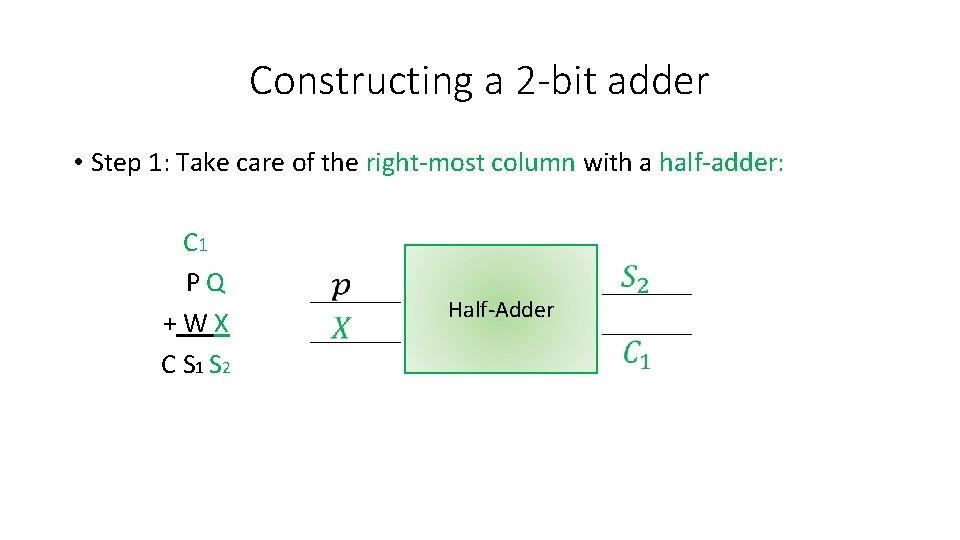Constructing a 2 -bit adder • Step 1: Take care of the right-most column with a half-adder: C 1 P Q + W X C S 1 S 2 Half-Adder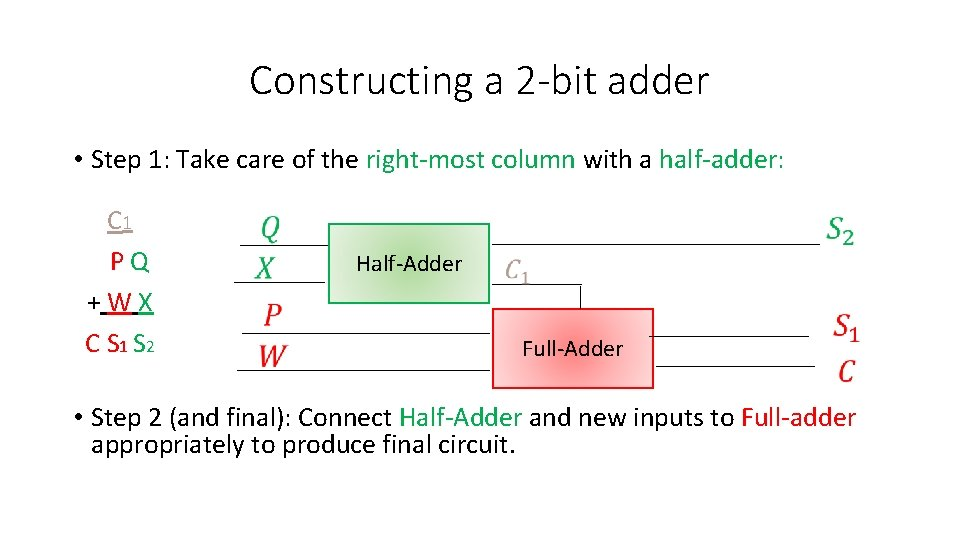Constructing a 2 -bit adder • Step 1: Take care of the right-most column with a half-adder: C 1 P Q + W X C S 1 S 2 Half-Adder Full-Adder • Step 2 (and final): Connect Half-Adder and new inputs to Full-adder appropriately to produce final circuit.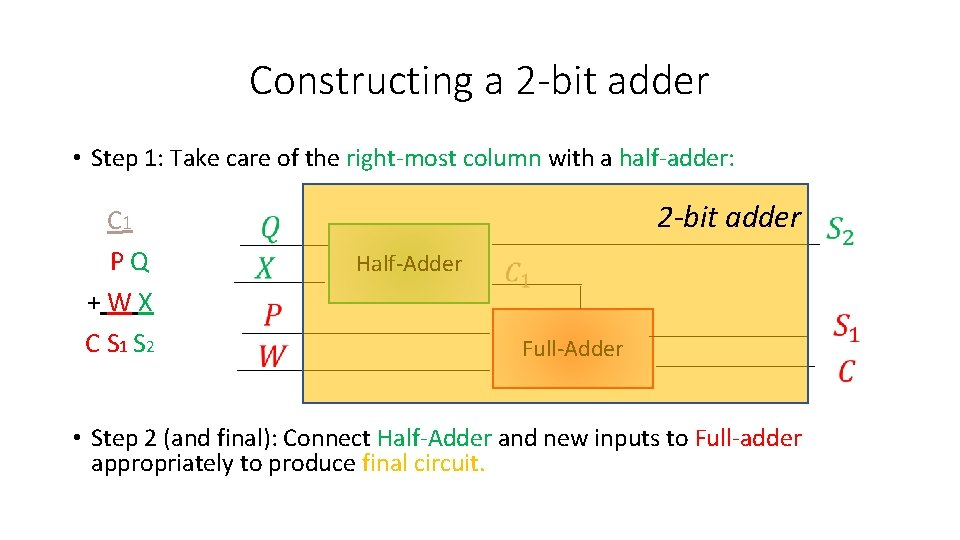Constructing a 2 -bit adder • Step 1: Take care of the right-most column with a half-adder: C 1 P Q + W X C S 1 S 2 2 -bit adder Half-Adder Full-Adder • Step 2 (and final): Connect Half-Adder and new inputs to Full-adder appropriately to produce final circuit.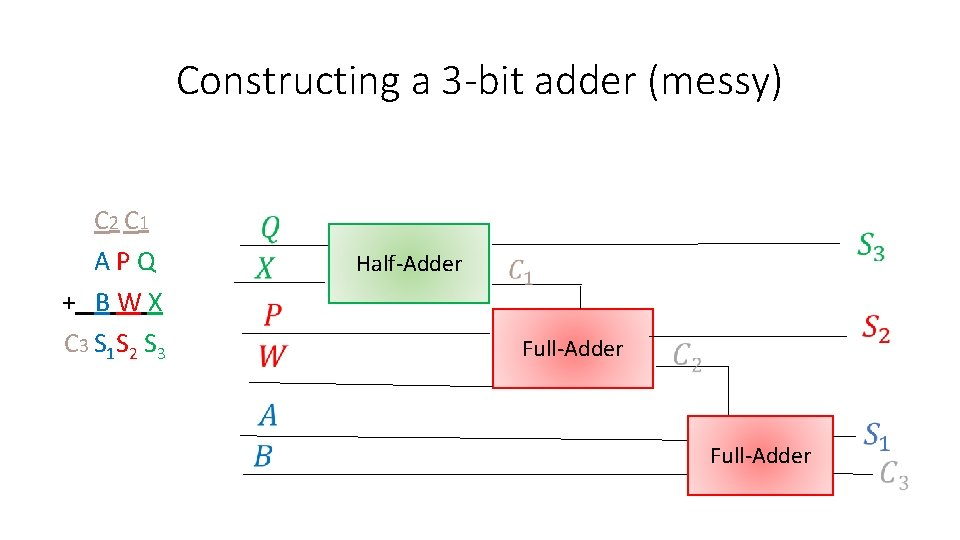Constructing a 3 -bit adder (messy) C 2 C 1 A P Q + B W X C 3 S 1 S 2 S 3 Half-Adder Full-Adder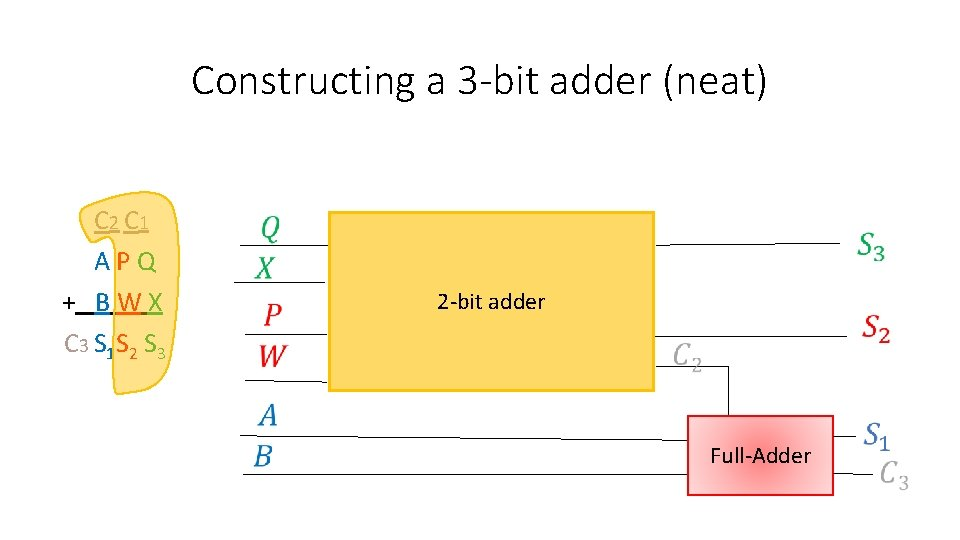Constructing a 3 -bit adder (neat) C 2 C 1 A P Q + B W X C 3 S 1 S 2 S 3 2 -bit adder Full-Adder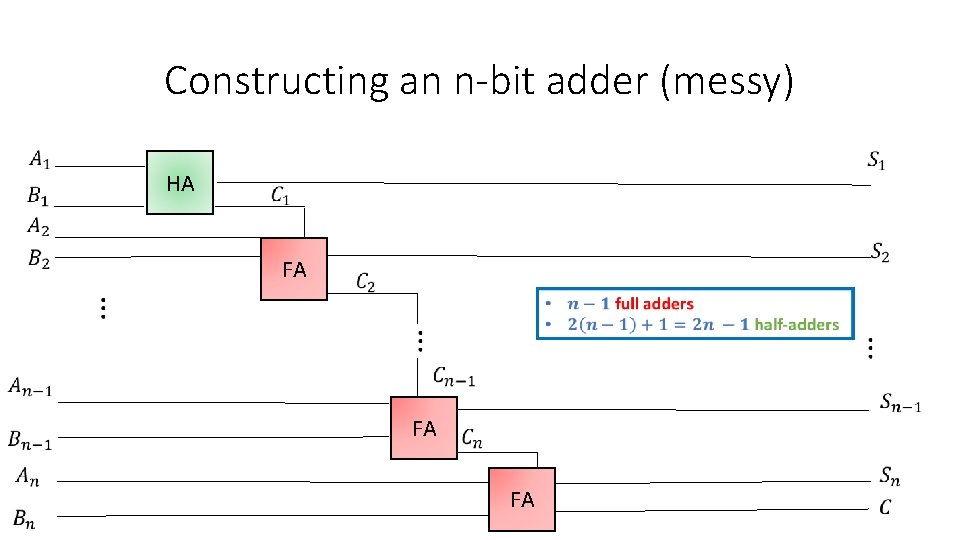Constructing an n-bit adder (messy) HA FA FA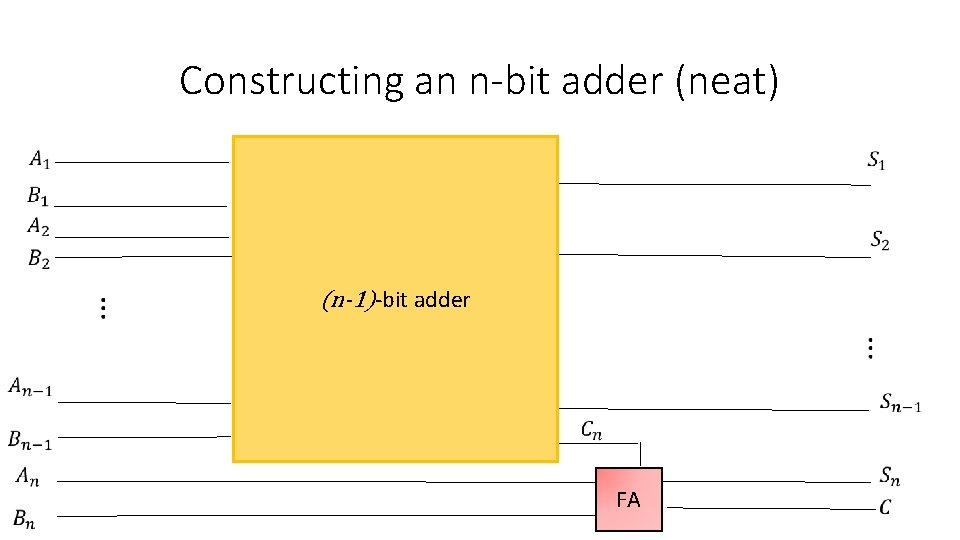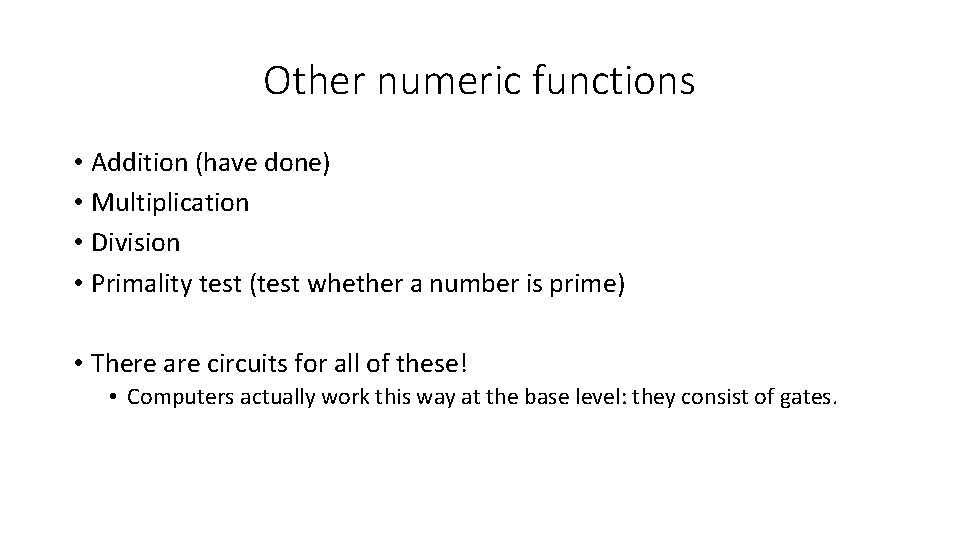Other numeric functions • Addition (have done) • Multiplication • Division • Primality test (test whether a number is prime) • There are circuits for all of these! • Computers actually work this way at the base level: they consist of gates.Fun exercise • Input: number in binary • Output: number in unary (!) 0 0 0 1 1 1 1 CircuitFirst micro-circuit 0 0 0 1 1 1 1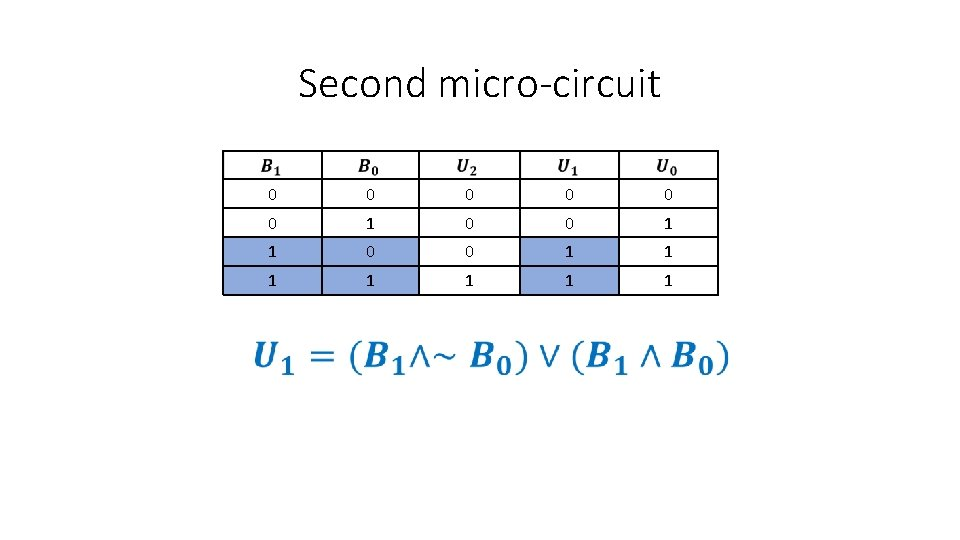Second micro-circuit 0 0 0 1 1 1 1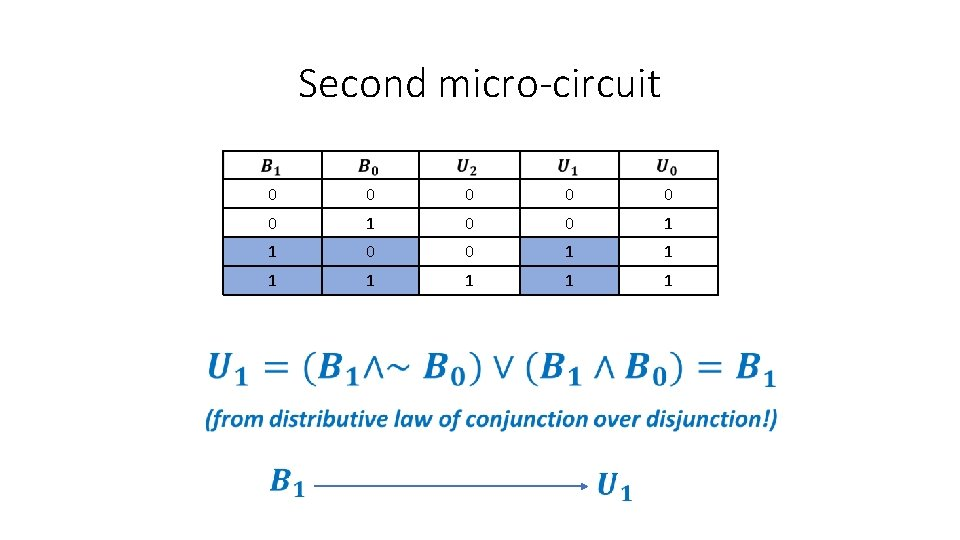Second micro-circuit 0 0 0 1 1 1 1Third micro-circuit 0 0 0 1 1 1 1Third micro-circuit 0 0 0 1 1 1 1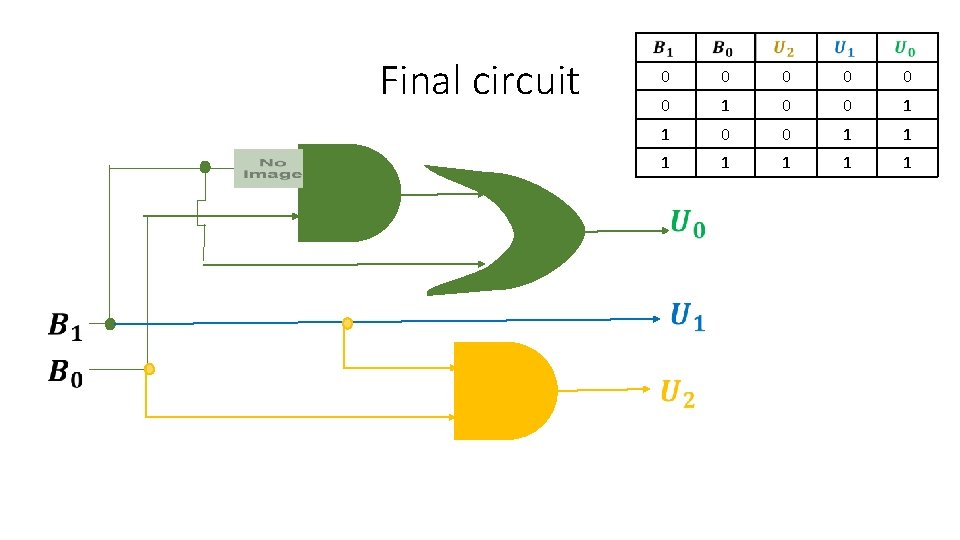Final circuit 0 0 0 1 1 1 1Final circuit 0 0 0 1 1 1 1 2 -to-3 binary to unary decoder!